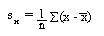## Title

Product Moment Correlation Coefficient
Quick revise

The product moment correlation coefficient is a measurement of the degree of scatter. It is usually denoted by r and r can be any value between -1 and 1. It is defined as follows:

r = sxy
sxsy

where sxy is the covariance of x and y,.

Correlation

The product moment correlation coefficient (pmcc) can be used to tell us how strong the correlation between two variables is.

A positive value indicates a positive correlation and the higher the value, the stronger the correlation. Similarly, a negative value indicates a negative correlation and the lower the value the stronger the correlation.

If there is a perfect positive correlation (in other words the points all lie on a straight line that goes up from left to right), then r = 1.

If there is a perfect negative correlation, then r = -1.

If there is no correlation, then r = 0. r would also be equal to zero if the variables were related in a non-linear way (they might lie on a quadratic curve rather than a straight line, for example).

Rate: